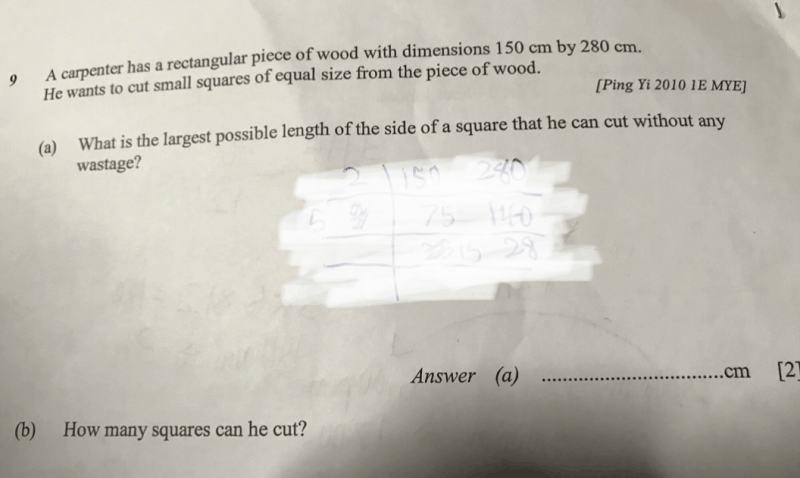# Questiona) The largest length of the square is simply the HCF of 150 and 280.

2 | 150 , 280
5 | 75, 140
– | 15, 28 (no more common factors)

HCF of 150 and 280 is 10. So the largest length is 10 cm.

b) 150 = 15 × 10
280 = 28 × 10

This means that we can cut out squares measuring 10 cm by 10 cm. There will be 28 of these squares across and 15 of these squares downwards, for a total of 28 × 15 =  420 squares.

0 Replies 1 Like ✔Accepted Answer

0 Replies 0 Likes